# Series and Parallel AC Circuits

## AC Electric Circuits

• #### Question 1

 Don’t just sit there! Build something!!

Learning to mathematically analyze circuits requires much study and practice. Typically, students practice by working through lots of sample problems and checking their answers against those provided by the textbook or the instructor. While this is good, there is a much better way.

You will learn much more by actually building and analyzing real circuits, letting your test equipment provide the “answers” instead of a book or another person. For successful circuit-building exercises, follow these steps:

1. Carefully measure and record all component values prior to circuit construction.
2. Draw the schematic diagram for the circuit to be analyzed.
3. Carefully build this circuit on a breadboard or other convenient medium.
4. Check the accuracy of the circuit’s construction, following each wire to each connection point, and verifying these elements one-by-one on the diagram.
5. Mathematically analyze the circuit, solving for all voltage and current values.
6. Carefully measure all voltages and currents, to verify the accuracy of your analysis.
7. If there are any substantial errors (greater than a few percent), carefully check your circuit’s construction against the diagram, then carefully re-calculate the values and re-measure.

For AC circuits where inductive and capacitive reactances (impedances) are a significant element in the calculations, I recommend high quality (high-Q) inductors and capacitors, and powering your circuit with low frequency voltage (power-line frequency works well) to minimize parasitic effects. If you are on a restricted budget, I have found that inexpensive electronic musical keyboards serve well as “function generators” for producing a wide range of audio-frequency AC signals. Be sure to choose a keyboard “voice” that closely mimics a sine wave (the “panflute” voice is typically good), if sinusoidal waveforms are an important assumption in your calculations.

As usual, avoid very high and very low resistor values, to avoid measurement errors caused by meter “loading”. I recommend resistor values between 1 kΩ and 100 kΩ.

One way you can save time and reduce the possibility of error is to begin with a very simple circuit and incrementally add components to increase its complexity after each analysis, rather than building a whole new circuit for each practice problem. Another time-saving technique is to re-use the same components in a variety of different circuit configurations. This way, you won’t have to measure any component’s value more than once.

• #### Question 2

Which component, the resistor or the capacitor, will drop more voltage in this circuit?Also, calculate the total impedance (Ztotal) of this circuit, expressing it in both rectangular and polar forms.

• #### Question 3

Determine the input frequency necessary to give the output voltage a phase shift of 40o: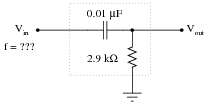• #### Question 4

Determine the input frequency necessary to give the output voltage a phase shift of -38o: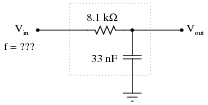• #### Question 5

Write an equation that solves for the impedance of this series circuit. The equation need not solve for the phase angle between voltage and current, but merely provide a scalar figure for impedance (in ohms):• #### Question 6

Draw a phasor diagram showing the trigonometric relationship between resistance, reactance, and impedance in this series circuit:Show mathematically how the resistance and reactance combine in series to produce a total impedance (scalar quantities, all). Then, show how to analyze this same circuit using complex numbers: regarding each of the component as having its own impedance, demonstrating mathematically how these impedances add up to comprise the total impedance (in both polar and rectangular forms).

• #### Question 7

Solve for all voltages and currents in this series RC circuit, and also calculate the phase angle of the total impedance:• #### Question 8

A student is asked to calculate the phase shift for the following circuit’s output voltage, relative to the phase of the source voltage:He recognizes this as a series circuit, and therefore realizes that a right triangle would be appropriate for representing component impedances and component voltage drops (because both impedance and voltage are quantities that add in series, and the triangle represents phasor addition):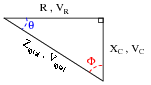The problem now is, which angle does the student solve for in order to find the phase shift of Vout? The triangle contains two angles besides the 90o angle, Θ and Φ. Which one represents the output phase shift, and more importantly, why?

• #### Question 9

Calculate the output voltage of this phase-shifting circuit, expressing it in polar form (magnitude and phase angle relative to the source voltage):• #### Question 10

Calculate the output voltage of this phase-shifting circuit, expressing it in polar form (magnitude and phase angle relative to the source voltage):• #### Question 11

In this circuit, a series resistor-capacitor network creates a phase-shifted voltage for the “gate” terminal of a power-control device known as a TRIAC. All portions of the circuit except for the RC network are “shaded” for de-emphasis: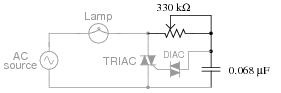Calculate how many degrees of phase shift the capacitor’s voltage is, compared to the total voltage across the series RC network, assuming a frequency of 60 Hz, and a 50% potentiometer setting.

• #### Question 12

Determine the input frequency necessary to give the output voltage a phase shift of 70o: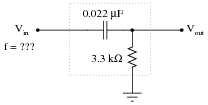• #### Question 13

Determine the input frequency necessary to give the output voltage a phase shift of -25o: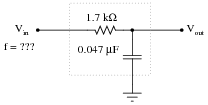• #### Question 14

Write an equation that solves for the impedance of this series circuit. The equation need not solve for the phase angle between voltage and current, but merely provide a scalar figure for impedance (in ohms):• #### Question 15

Draw a phasor diagram showing the trigonometric relationship between resistance, reactance, and impedance in this series circuit:Show mathematically how the resistance and reactance combine in series to produce a total impedance (scalar quantities, all). Then, show how to analyze this same circuit using complex numbers: regarding component as having its own impedance, demonstrating mathematically how these impedances add up to comprise the total impedance (in both polar and rectangular forms).

• #### Question 16

Calculate the total impedance for these two 100 mH inductors at 2.3 kHz, and draw a phasor diagram showing circuit impedances (Ztotal, R, and X):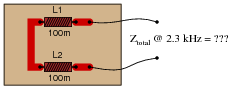Now, re-calculate impedance and re-draw the phasor impedance diagram supposing the second inductor is replaced by a 1.5 kΩ resistor: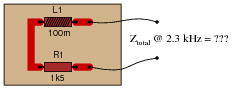• #### Question 17

Calculate the total impedance of this series LR circuit and then calculate the total circuit current:Also, draw a phasor diagram showing how the individual component impedances relate to the total impedance.

• #### Question 18

Calculate the magnitude and phase shift of the current through this inductor, taking into consideration its intrinsic winding resistance:• #### Question 19

Solve for all voltages and currents in this series LR circuit:• #### Question 20

Solve for all voltages and currents in this series LR circuit, and also calculate the phase angle of the total impedance:• #### Question 21

Determine the total current and all voltage drops in this circuit, stating your answers the way a multimeter would register them: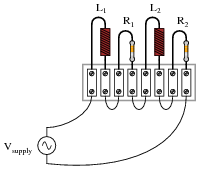L1 = 250 mH
L2 = 60 mH
R1 = 6.8 kΩ
R2 = 1.2 kΩ
Vsupply = 13.4 V RMS
fsupply = 6.5 kHz

Also, calculate the phase angle (Θ) between voltage and current in this circuit, and explain where and how you would connect an oscilloscope to measure that phase shift.

• #### Question 22

One way to vary the amount of power delivered to a resistive AC load is by varying another resistance connected in series:A problem with this power control strategy is that power is wasted in the series resistance (I2Rseries). A different strategy for controlling power is shown here, using a series inductance rather than resistance:Explain why the latter circuit is more power-efficient than the former, and draw a phasor diagram showing how changes in Lseries affect Ztotal.

• #### Question 23

A quantity sometimes used in DC circuits is conductance, symbolized by the letter G. Conductance is the reciprocal of resistance (G = 1/R), and it is measured in the unit of siemens.

Expressing the values of resistors in terms of conductance instead of resistance has certain benefits in parallel circuits. Whereas resistances (R) add in series and “diminish” in parallel (with a somewhat complex equation), conductances (G) add in parallel and “diminish” in series. Thus, doing the math for series circuits is easier using resistance and doing math for parallel circuits is easier using conductance:In AC circuits, we also have reciprocal quantities to reactance (X) and impedance (Z). The reciprocal of reactance is called susceptance (B = 1/X), and the reciprocal of impedance is called admittance (Y = 1/Z). Like conductance, both these reciprocal quantities are measured in units of siemens.

Write an equation that solves for the admittance (Y) of this parallel circuit. The equation need not solve for the phase angle between voltage and current, but merely provide a scalar figure for admittance (in siemens):• #### Question 24

Calculate the total impedance for these two 100 mH inductors at 2.3 kHz, and draw a phasor diagram showing circuit admittances (Ytotal, G, and B):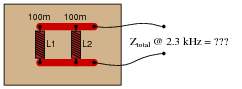Now, re-calculate impedance and re-draw the phasor admittance diagram supposing the second inductor is replaced by a 1.5 kΩ resistor: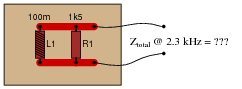• #### Question 25

Calculate the individual currents through the inductor and through the resistor, the total current, and the total circuit impedance:Also, draw a phasor diagram showing how the individual component currents relate to the total current.

• #### Question 26

A large AC electric motor under load can be considered as a parallel combination of resistance and inductance:Calculate the current necessary to power this motor if the equivalent resistance and inductance is 20 Ω and 238 mH, respectively.

• #### Question 27

A large AC electric motor under load can be considered as a parallel combination of resistance and inductance:Calculate the equivalent inductance (Leq) if the measured source current is 27.5 amps and the motor’s equivalent resistance (Req) is 11.2 Ω.

• #### Question 28

Determine the total current and all component currents in this circuit, stating your answers the way a multimeter would register them: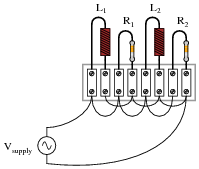L1 = 1.2 H
L2 = 650 mH
R1 = 33 kΩ
R2 = 27 kΩ
Vsupply = 19.7 V RMS
fsupply = 4.5 kHz

Also, calculate the phase angle (Θ) between voltage and current in this circuit, and explain where and how you would connect an oscilloscope to measure that phase shift.

• #### Question 29

Calculate the total impedances (complete with phase angles) for each of the following inductor-resistor circuits:• #### Question 30

A doorbell ringer has a solenoid with an inductance of 63 mH connected in parallel with a lamp (for visual indication) having a resistance of 150 ohms:Calculate the phase shift of the total current (in units of degrees) in relation to the total supply voltage, when the doorbell switch is actuated.

• #### Question 31

An AC electric motor operating under loaded conditions draws a current of 11 amps (RMS) from the 120 volt (RMS) 60 Hz power lines. The measured phase shift between voltage and current for this motor is 34o, with voltage leading current.

Determine the equivalent parallel combination of resistance (R) and inductance (L) that is electrically equivalent to this operating motor.

• #### Question 32

Doorbell circuits connect a small lamp in parallel with the doorbell pushbutton so that there is light at the button when it is not being pressed. The lamp’s filament resistance is such that there is not enough current going through it to energize the solenoid coil when lit, which means the doorbell will ring only when the pushbutton switch shorts past the lamp:Suppose that such a doorbell circuit suddenly stops working one day, and the home owner assumes the power source has quit since the bell will not ring when the button is pressed and the lamp never lights. Although a dead power source is certainly possible, it is not the only possibility. Identify another possible failure in this circuit which would result in no doorbell action (no sound) and no light at the lamp.

• #### Question 33

Calculate the total impedance offered by these two inductors to a sinusoidal signal with a frequency of 60 Hz: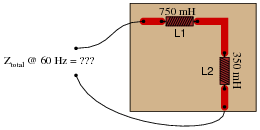Show your work using two different problem-solving strategies:

Calculating total inductance (Ltotal) first, then total impedance (Ztotal).
Calculating individual impedances first (ZL1 and ZL2), then total impedance (Ztotal).

Do these two strategies yield the same total impedance value? Why or why not?

• #### Question 34

Calculate the total impedance of this LR circuit, once using nothing but scalar numbers, and again using complex numbers: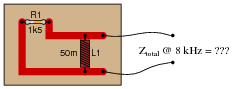• #### Question 35

Calculate the total impedance offered by these two inductors to a sinusoidal signal with a frequency of 120 Hz: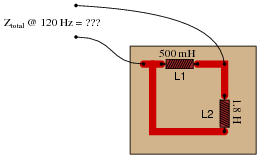Show your work using three different problem-solving strategies:

Calculating total inductance (Ltotal) first, then total impedance (Ztotal).
Calculating individual admittances first (YL1 and YL2), then total admittance (Ytotal), then total impedance (Ztotal).
Using complex numbers: calculating individual impedances first (ZL1 and ZL2), then total impedance (Ztotal).

Do these two strategies yield the same total impedance value? Why or why not?

• #### Question 36

Determine the input frequency necessary to give the output voltage a phase shift of 75o: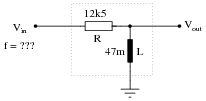Also, write an equation that solves for frequency (f), given all the other variables (R, L, and phase angle θ).

• #### Question 37

Determine the necessary resistor value to give the output voltage a phase shift of 44o: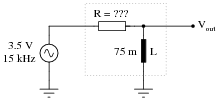Also, write an equation that solves for this resistance value (R), given all the other variables (f, L, and phase angle θ).

• #### Question 38

Determine the input frequency necessary to give the output voltage a phase shift of -40o: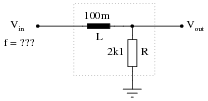Also, write an equation that solves for frequency (f), given all the other variables (R, L, and phase angle θ).

• #### Question 39

Determine the necessary resistor value to give the output voltage a phase shift of -60o: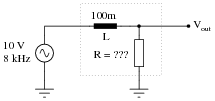Also, write an equation that solves for this resistance value (R), given all the other variables (f, L, and phase angle θ).

• #### Question 40

Calculate the necessary size of the capacitor to give this circuit a total impedance (Ztotal) of 4 kΩ, at a power supply frequency of 100 Hz:• #### Question 41

Capacitors and inductors are complementary components - both conceptually and mathematically, they seem to be almost exact opposites of each other. Calculate the total impedance of this series-connected inductor and capacitor network:• #### Question 42

Write an equation that solves for the impedance of this series circuit. The equation need not solve for the phase angle between voltage and current, but merely provide a scalar figure for impedance (in ohms):• #### Question 43

Write an equation that solves for the admittance of this parallel circuit. The equation need not solve for the phase angle between voltage and current, but merely provide a scalar figure for admittance (in siemens):• #### Question 44

Calculate the total impedance of this parallel network, given a signal frequency of 12 kHz:• #### Question 45

Is this circuit’s overall behavior capacitive or inductive? In other words, from the perspective of the AC voltage source, does it “appear” as though a capacitor is being powered, or an inductor?Now, suppose we take these same components and re-connect them in parallel rather than series. Does this change the circuit’s overall “appearance” to the source? Does the source now “see” an equivalent capacitor or an equivalent inductor? Explain your answer.• #### Question 46

Suppose you are building a circuit and you need an impedance of 1500 Ω ∠ -41o at a frequency of 600 Hz. What combination of components could you connect together in series to achieve this precise impedance?

• #### Question 47

Calculate the impedance of a 145 mH inductor connected in series with 750 Ω resistor at a frequency of 1 kHz, then determine the necessary resistor and inductor values to create the exact same total impedance in a parallel configuration.

• #### Question 48

Calculate the total impedance of these parallel-connected components, expressing it in polar form (magnitude and phase angle):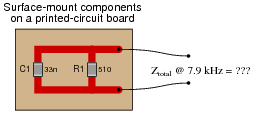Also, draw an admittance triangle for this circuit.

• #### Question 49

Determine the necessary resistor value to give the output voltage a phase shift of -64o: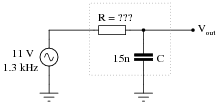Also, write an equation that solves for this resistance value (R), given all the other variables (f, C, and phase angle θ).

• #### Question 50

Calculate the total impedance offered by these two capacitors to a sinusoidal signal with a frequency of 3 kHz:Show your work using two different problem-solving strategies:

Calculating total capacitance (Ctotal) first, then total impedance (Ztotal).
Calculating individual impedances first (ZC1 and ZC2), then total impedance (Ztotal).

Do these two strategies yield the same total impedance value? Why or why not?

• #### Question 51

A student measures voltage drops in an AC circuit using three voltmeters and arrives at the following measurements: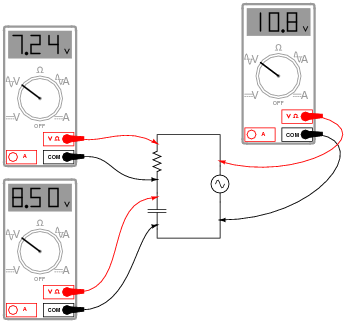Upon viewing these measurements, the student becomes very perplexed. Aren’t voltage drops supposed to add in series, just as in DC circuits? Why, then, is the total voltage in this circuit only 10.8 volts and not 15.74 volts? How is it possible for the total voltage in an AC circuit to be substantially less than the simple sum of the components’ voltage drops?

Another student, trying to be helpful, suggests that the answer to this question might have something to do with RMS versus peak measurements. A third student disagrees, proposing instead that at least one of the meters is badly out of calibration and thus not reading correctly.

When you are asked for your thoughts on this problem, you realize that neither of the answers proposed thus far are correct. Explain the real reason for the “discrepancy” in voltage measurements, and also explain how you could experimentally disprove the other answers (RMS vs. peak, and bad calibration).

• #### Question 52

A quantity sometimes used in DC circuits is conductance, symbolized by the letter G. Conductance is the reciprocal of resistance (G = 1/R), and it is measured in the unit of siemens.

Expressing the values of resistors in terms of conductance instead of resistance has certain benefits in parallel circuits. Whereas resistances (R) add in series and “diminish” in parallel (with a somewhat complex equation), conductances (G) add in parallel and “diminish” in series. Thus, doing the math for series circuits is easier using resistance and doing math for parallel circuits is easier using conductance:In AC circuits, we also have reciprocal quantities to reactance (X) and impedance (Z). The reciprocal of reactance is called susceptance (B = 1/X), and the reciprocal of impedance is called admittance (Y = 1/Z). Like conductance, both these reciprocal quantities are measured in units of siemens.

Write an equation that solves for the admittance (Y) of this parallel circuit. The equation need not solve for the phase angle between voltage and current, but merely provide a scalar figure for admittance (in siemens):• #### Question 53

Calculate the total impedance offered by these three resistors to a sinusoidal signal with a frequency of 10 kHz:

R1 = 3.3 kΩ
R2 = 10 kΩ
R3 = 5 kΩ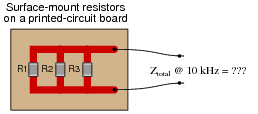State your answer in the form of a scalar number (not complex), but calculate it using two different strategies:

Calculate total resistance (Rtotal) first, then total impedance (Ztotal).
Calculate individual admittances first (YR1, YR2, and YR3), then total impedance (Ztotal).
• #### Question 54

Calculate the total impedance offered by these three capacitors to a sinusoidal signal with a frequency of 4 kHz:

C1 = 0.1 μF
C2 = 0.047 μF
C3 = 0.033 μF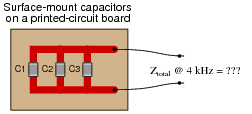State your answer in the form of a scalar number (not complex), but calculate it using two different strategies:

Calculate total capacitance (Ctotal) first, then total impedance (Ztotal).
Calculate individual admittances first (YC1, YC2, and YC3), then total impedance (Ztotal).
• #### Question 55

Calculate the total impedance of this RC circuit, once using nothing but scalar numbers, and again using complex numbers: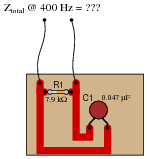• #### Question 56

Calculate the total impedance offered by these two capacitors to a sinusoidal signal with a frequency of 900 Hz:Show your work using three different problem-solving strategies:

Calculating total capacitance (Ctotal) first, then total impedance (Ztotal).
Calculating individual admittances first (YC1 and YC2), then total admittance (Ytotal), then total impedance (Ztotal).
Using complex numbers: calculating individual impedances first (ZC1 and ZC2), then total impedance (Ztotal).

Do these two strategies yield the same total impedance value? Why or why not?

• #### Question 57

Due to the effects of a changing electric field on the dielectric of a capacitor, some energy is dissipated in capacitors subjected to AC. Generally, this is not very much, but it is there. This dissipative behavior is typically modeled as a series-connected resistance:Calculate the magnitude and phase shift of the current through this capacitor, taking into consideration its equivalent series resistance (ESR):Compare this against the magnitude and phase shift of the current for an ideal 0.22 μF capacitor.

• #### Question 58

Solve for all voltages and currents in this series RC circuit:• #### Question 59

A technician needs to know the value of a capacitor, but does not have a capacitance meter nearby. In lieu of this, the technician sets up the following circuit to measure capacitance: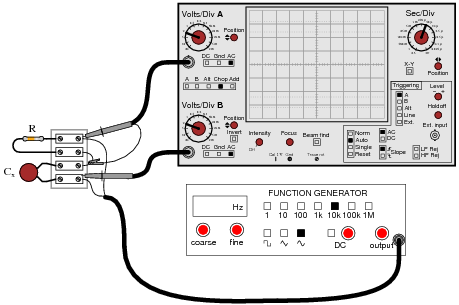You happen to walk by this technician’s workbench and ask, “How does this measurement setup work?” The technician responds, “You connect a resistor of known value (R) in series with the capacitor of unknown value (Cx), then adjust the generator frequency until the oscilloscope shows the two voltage drops to be equal, and then you calculate Cx.”

Explain how this system works, in your own words. Also, write the formula you would use to calculate the value of Cx given f and R.

• #### Question 60

If the dielectric substance between a capacitor’s plates is not a perfect insulator, there will be a path for direct current (DC) from one plate to the other. This is typically called leakage resistance, and it is modeled as a shunt resistance to an ideal capacitance:Calculate the magnitude and phase shift of the current drawn by this real capacitor, if powered by a sinusoidal voltage source of 30 volts RMS at 400 Hz:Compare this against the magnitude and phase shift of the current for an ideal capacitor (no leakage).

• #### Question 61

Voltage divider circuits may be constructed from reactive components just as easily as they may be constructed from resistors. Take this capacitive voltage divider, for instance:Calculate the magnitude and phase shift of Vout. Also, describe what advantages a capacitive voltage divider might have over a resistive voltage divider.

• #### Question 62

Determine the input frequency necessary to give the output voltage a phase shift of 25o: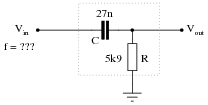Also, write an equation that solves for frequency (f), given all the other variables (R, C, and phase angle θ).

• #### Question 63

Determine the necessary resistor value to give the output voltage a phase shift of 58o: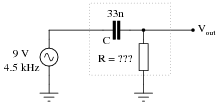Also, write an equation that solves for this resistance value (R), given all the other variables (f, C, and phase angle θ).

• #### Question 64

Use algebraic substitution to generate an equation expressing the output voltage of the following circuit given the input voltage, the input frequency, the capacitor value, and the resistor value:Vout =

• #### Question 65

Use algebraic substitution to generate an equation expressing the output voltage of the following circuit given the input voltage, the input frequency, the capacitor value, and the resistor value:Vout =

• #### Question 66

Determine the total current and all voltage drops in this circuit, stating your answers the way a multimeter would register them: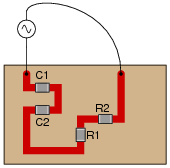C1 = 125 pF
C2 = 71 pF
R1 = 6.8 kΩ
R2 = 1.2 kΩ
Vsupply = 20 V RMS
fsupply = 950 kHz

Also, calculate the phase angle (Θ) between voltage and current in this circuit, and explain where and how you would connect an oscilloscope to measure that phase shift.

• #### Question 67

Calculate the voltage drops across all components in this circuit, expressing them in complex (polar) form with magnitudes and phase angles each: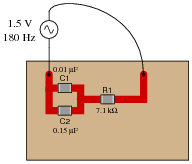• #### Question 68

Determine the phase angle (Θ) of the current in this circuit, with respect to the supply voltage: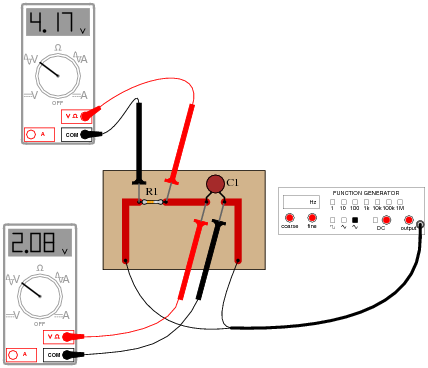• #### Question 69

Calculate the total (source) current in this parallel RC circuit, based on the readings taken by the dual-trace oscilloscope (both of the inductive current probes show branch currents in units of 1 amp per division):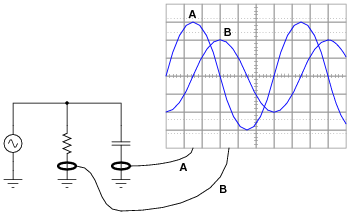Itotal =

• #### Question 70

Calculate the total impedances (complete with phase angles) for each of the following capacitor-resistor circuits:• #### Question 71

Complete the table of values for this circuit, representing all quantities in complex-number form (either polar or rectangular, your choice):• #### Question 72

Determine the size of capacitor (in Farads) necessary to create a total current of 11.3 mA in this parallel RC circuit:• #### Question 73

The input impedance of an electrical test instrument is a very important parameter in some applications, because of how the instrument may load the circuit being tested. Oscilloscopes are no different from voltmeters in this regard: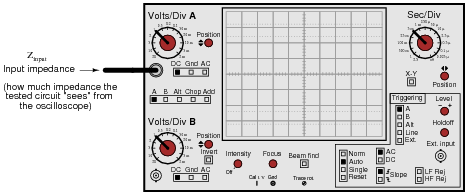Typical input impedance for an oscilloscope is 1 MΩ of resistance, in parallel with a small amount of capacitance. At low frequencies, the reactance of this capacitance is so high that it may be safely ignored. At high frequencies, though, it may become a substantial load to the circuit under test: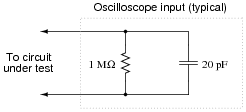Calculate how many ohms of impedance this oscilloscope input (equivalent circuit shown in the above schematic) will impose on a circuit with a signal frequency of 150 kHz.

• #### Question 74

If the source voltage in this circuit is assumed to be the phase reference (that is, the voltage is defined to be at an angle of 0 degrees), determine the relative phase angles of each current in this parallel circuit: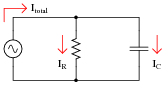ΘI(R) =
ΘI(C) =
ΘI(total) =
• #### Question 75

Calculate the voltage dropped across the inductor, the capacitor, and the 8-ohm speaker in this sound system at the following frequencies, given a constant source voltage of 15 volts:f = 200 Hz
f = 550 Hz
f = 900 Hz

Regard the speaker as nothing more than an 8-ohm resistor.